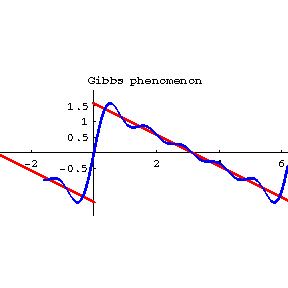Gibbs phenomenon

A characteristic of the behaviour of the partial sums (or their averages) of a Fourier series.Figure: g044410a

First noted by H. Wilbraham  and rediscovered by J.W. Gibbs  at a much later date. Let the partial sums $s _ {n}$ of the Fourier series of a function $f$ converge to $f$ in some neighbourhood $\{ {x } : {0 < | x - x _ {0} | < h } \}$ of a point $x _ {0}$ at which

$$a \equiv f( x _ {0} - ) \leq f ( x _ {0} + ) \equiv b.$$

The Gibbs phenomenon takes place for $s _ {n}$ at $x _ {0}$ if $A < a \leq b < B$ where

$$A = \ {fnnme \underline{lim} } _ {\begin{array}{c} n \rightarrow \infty \\ x \uparrow x _ {0} \end{array} } \ s _ {n} ( x),$$

$$B = \overline{\lim\limits}\; _ {\begin{array}{c} n \rightarrow \infty \\ x \downarrow x _ {0} \end{array} } s _ {n} ( x).$$

The geometrical meaning of this is that the graphs (cf. Fig.) of the partial sums $s _ {n}$ do not approach the "expected" interval $[ a, b ]$ on the vertical line $x = x _ {0}$, but approach the strictly-larger interval $[ A, B ]$ as $x \rightarrow x _ {0}$ and $n \rightarrow \infty$. The Gibbs phenomenon is defined in an analogous manner for averages of the partial sums of a Fourier series when the latter is summed by some given method.

For instance, the following theorems are valid for $2 \pi$- periodic functions $f$ of bounded variation on $[ - \pi , \pi ]$.

1) At points of non-removable discontinuity, and only at such points, the Gibbs phenomenon occurs for $s _ {n}$. In particular, if $f( x) = ( \pi - x)/2$ for $0 < x < 2 \pi$, then for the point $x = 0$ the segment $[ a, b] = [- \pi /2 , \pi /2]$, while the segment $[ A, B] = [- l, l]$ where

$$l = \int\limits _ { 0 } ^ \pi \frac{\sin t }{t } \ dt \approx 1.85 \dots > { \frac \pi {2} } .$$

2) There exists an absolute constant $\alpha _ {0}$, $0 < \alpha _ {0} < 1$, such that the Cesàro averages $\sigma _ {n} ^ \alpha$ do not have the Gibbs phenomenon if $\alpha \geq \alpha _ {0}$, while if $\alpha < \alpha _ {0}$ the phenomenon is observed at all points of non-removable discontinuity of $f$.

How to Cite This Entry:
Gibbs phenomenon. Encyclopedia of Mathematics. URL: http://encyclopediaofmath.org/index.php?title=Gibbs_phenomenon&oldid=47097
This article was adapted from an original article by P.L. Ul'yanov (originator), which appeared in Encyclopedia of Mathematics - ISBN 1402006098. See original article